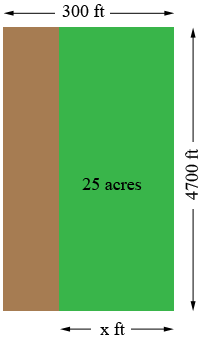SEARCH HOMEMath Central Quandaries & QueriesQuestion from Va, hello, can you pls help me....i have a property which has 300 ft width road frontage and my lot is 4700 ft deep to the back line of my property which i am told 27 acres. I want to only sell 25 acres...and go to the rear line with 4700 feet, but decrease the front width using the 300 ft measurement. how do i decrease the 300 ft width measurement by 2 acres? i am confused what the measurement would be..thank you....so to sum it i want to make 25 acres out of a measurement of 300 x 4700 ft long reducing the front measurement..thank you so much.Hi,

If your property is a rectangle which is 300 feet by 4700 feet and you want to cut off a strip of with $x$ feet and area of 25 acres then the diagram would look something like this.There are 43,560 square feet in an acre so 25 acres is $25 \times 43560$ square feet. Thus

$25 \times 43560 = 4700 \times x$

or $x = \frac{25 \times 43560}{4700} \mbox{ feet.}$

PennyMath Central is supported by the University of Regina and The Pacific Institute for the Mathematical Sciences.